Next: Differential Calculus Up: Topics to Study Previous: Elementary Functions

## Limits

Properties of Limits

If b and c are real numbers, n is a positive integer, and the functions ƒ and g have limits as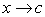, then the following properties are true.

 1. Scalar multiple: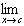[b(ƒ(x))] = b[ƒ(x)] 2. Sum or difference:[ƒ(x)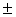g(x)] =ƒ(x)g(x) 3. Product:[ƒ(x)g(x)] = [ƒ(x)][g(x)] 4. Quotient:[ƒ(x)/g(x)] = [ƒ(x)]/[g(x)], ifg(x)0

One-Sided Limits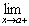ƒ(x) x approaches c from the right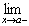ƒ(x) x approaches c from the left

Limits at Infinityƒ(x) = L or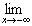ƒ(x) = L

The value of ƒ(x) approaches L as x increases/decreases without bound.

y = L is the horizontal asymptote of the graph of ƒ.

Some Nonexistent Limits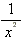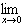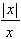sin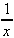Some Infinite Limits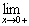ln x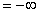Exercise: What is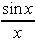? (A) 1 (B) 0 (C)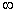(D)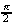(E) The limit does not exist. /TD> The answer is A. You should memorize this limit.

Continuity

Definition

A function ƒ is continuous at c if:

1. ƒ(c) is defined

2.ƒ(x) exists

3.ƒ(x) = ƒ(c)

Graphically, the function is continuous at c if a pencil can be moved along the graph of ƒ(x) through (c, ƒ(c)) without lifting it off the graph.

 Exercise: If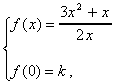for x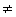0 and if ƒ is continuous at x = 0, then k = (A) -3/2 (B) -1 (C) 0 (D) 1 (E) 3/2 The answer is E.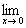ƒ(x) = 3/2

Intermediate Value Theorem

If ƒ is continuous on [a, b] and k is any number between ƒ(a) and ƒ(b), then there is at least one number c between a and b such that ƒ(c) = k.Next: Differential Calculus Up: Topics to Study Previous: Elementary Functions